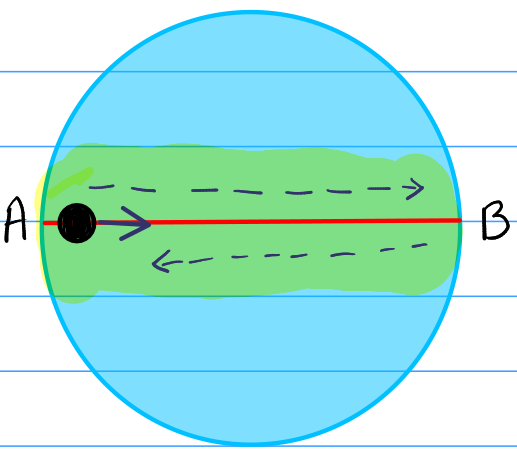How Cheenta works to ensure student success?
Explore the Back-Story

# NSEP 2015 Problem 12 | Periodic Motion due to Electrostatic ForceTry this problem on periodic motion due to electrostatic force from NSEP 2015 Problem 12.

## NSEP 2015-16 Problem 12

A particle of mass m and charge $-q$ moves along a diameter of a uniform spherical distribution of radius R with total charge $+Q$. The angular frequency of the periodic motion performed by the particle is

a) $\sqrt{\frac{2\pi qQ}{\epsilon_0 mR^3}}$ b) $\sqrt{\frac{qQ}{2\pi \epsilon_0 mR^3}}$ c) $\sqrt{\frac{qQ}{\epsilon_0 mR^3}}$ d) $\sqrt{\frac{qQ}{4\pi \epsilon_0 mR^3}}$

### Key Concepts

Periodic Motion

Gauss's Law of Electrostatic

## Suggested Book | Source | Answer

Concept of Physics H.C. Verma

University Physics by H. D. Young and R.A. Freedman

Fundamental of Physics D. Halliday, J. Walker and R. Resnick

National Standard Examination in Physics(NSEP) 2015-2016

Option-(d) $\sqrt{\frac{qQ}{4\pi \epsilon_0 mR^3}}$

## Try with Hints

The problem describe a structure something like that,

The whole blue region is charged and the black particle oscillates between A and B. We have to find the angular velocity of this oscillation.

To solve this problem we have to find what will be the Electric force inside the sphere. Using the gauss's law we can solve that.

Using the gauss's law, if we consider a Gaussian surface (sphere) of radius $r$, then charge inside that region is $Q_{in} = \frac{Q}{\frac{4}{3}\pi R^3}\frac{4}{3}\pi r^3 = Q\cdot \frac{r^3}{R^3}$

$$E\cdot 4\pi r^2 = \frac{Q_{in}}{\epsilon_0}$$

This means the particle will get a force of $F = -q E = -\frac{qQ}{4\pi \epsilon}\frac{r}{R^3}$

Arranging this in a equation,

$$F = m\frac{d^2r}{dt^2} = -\frac{qQr}{4\pi \epsilon R^3}$$

This reduces to ,

$$\frac{d^2r}{dt^2} + \frac{qQ}{4\pi \epsilon mR^3}r =0$$

We know that $w^2 = \frac{qQ}{4\pi \epsilon mR^3}$. This gives $$w = \sqrt{\frac{qQ}{4\pi \epsilon_0 mR^3}}$$

## Subscribe to Cheenta at Youtube

Try this problem on periodic motion due to electrostatic force from NSEP 2015 Problem 12.

## NSEP 2015-16 Problem 12

A particle of mass m and charge $-q$ moves along a diameter of a uniform spherical distribution of radius R with total charge $+Q$. The angular frequency of the periodic motion performed by the particle is

a) $\sqrt{\frac{2\pi qQ}{\epsilon_0 mR^3}}$ b) $\sqrt{\frac{qQ}{2\pi \epsilon_0 mR^3}}$ c) $\sqrt{\frac{qQ}{\epsilon_0 mR^3}}$ d) $\sqrt{\frac{qQ}{4\pi \epsilon_0 mR^3}}$

### Key Concepts

Periodic Motion

Gauss's Law of Electrostatic

## Suggested Book | Source | Answer

Concept of Physics H.C. Verma

University Physics by H. D. Young and R.A. Freedman

Fundamental of Physics D. Halliday, J. Walker and R. Resnick

National Standard Examination in Physics(NSEP) 2015-2016

Option-(d) $\sqrt{\frac{qQ}{4\pi \epsilon_0 mR^3}}$

## Try with Hints

The problem describe a structure something like that,

The whole blue region is charged and the black particle oscillates between A and B. We have to find the angular velocity of this oscillation.

To solve this problem we have to find what will be the Electric force inside the sphere. Using the gauss's law we can solve that.

Using the gauss's law, if we consider a Gaussian surface (sphere) of radius $r$, then charge inside that region is $Q_{in} = \frac{Q}{\frac{4}{3}\pi R^3}\frac{4}{3}\pi r^3 = Q\cdot \frac{r^3}{R^3}$

$$E\cdot 4\pi r^2 = \frac{Q_{in}}{\epsilon_0}$$

This means the particle will get a force of $F = -q E = -\frac{qQ}{4\pi \epsilon}\frac{r}{R^3}$

Arranging this in a equation,

$$F = m\frac{d^2r}{dt^2} = -\frac{qQr}{4\pi \epsilon R^3}$$

This reduces to ,

$$\frac{d^2r}{dt^2} + \frac{qQ}{4\pi \epsilon mR^3}r =0$$

We know that $w^2 = \frac{qQ}{4\pi \epsilon mR^3}$. This gives $$w = \sqrt{\frac{qQ}{4\pi \epsilon_0 mR^3}}$$

## Subscribe to Cheenta at Youtube

This site uses Akismet to reduce spam. Learn how your comment data is processed.

### Knowledge Partner